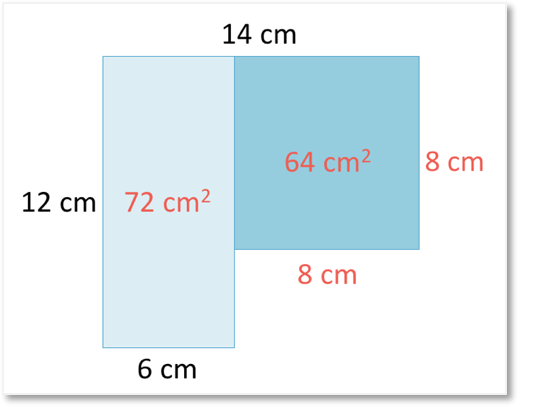# How to Find the Area of a Parallelogram

How to Find the Area of a Parallelogram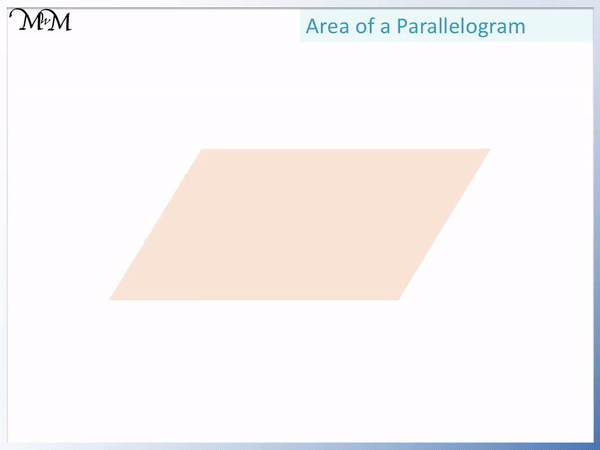• The area of a rectangle is length × width, which can also be written as base × height.
• A parallelogram is simply a rearranged rectangle.
• We can see that by taking a triangle out of one side of the rectangle and moving it to the other side, we get a parallelogram.
• The area is still the same, so the area of a parallelogram is base × height.
• The base is the distance along the bottom of the parallelogram.
• The height is the vertical distance directly from the base to the top of the parallelogram.
• The height and base measurements must be at right angles to each other.
• We technically say that the area of a parallelogram is base × perpendicular height, which means that the height and base are at right angles to each other.
The area of a parallelogram is base × perpendicular height.• The area of a parallelogram is base × height.
• The base is the distance along the bottom, which is 11 cm.
• The height is the distance from the bottom to the top of the parallelogram, which is 6 cm.
• The area is 11 cm × 6 cm = 66 cm2.
• The units for area are cm2 because we multiplied cm by cm.# Area of a Parallelogram

## How to Find the Area of a Parallelogram

To find the area of a parallelogram, multiply the base by the height. The base is the length of one side and the height is the direct distance between the base side and the side opposite to it. The base and the height must be at right angles to each other.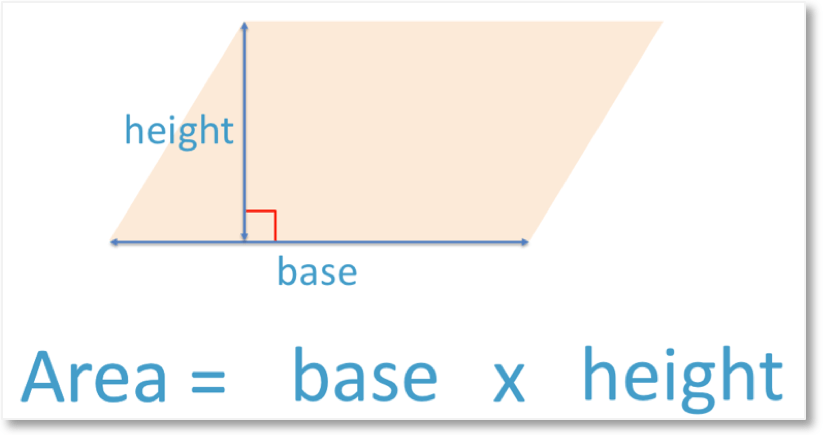The area of a parallelogram is calculated by multiplying the base and its perpendicular height. Perpendicular height tells us that the base and the height need to be at right angles to each other.

Here is an example of calculating the area of a parallelogram.The area of a parallelogram is base x height. The base and height are chosen as being two lengths that are at right angles to each other.

The base of a parallelogram is the outer side length, which runs along the bottom. Here the base is 11 cm.

The height of a parallelogram is the length between the base and the side opposite to the base. The height of this example is 6 cm.

The area is 11 cm x 6 cm = 66 cm2.Area is always written as units squared. Because the base and height lengths were measured in centimetres, the area is measured in centimetres squared, cm2.

Here is another example of finding the area of a parallelogram where the base is not on the bottom of the parallelogram.

We do not know the bottom-most side length of this shape.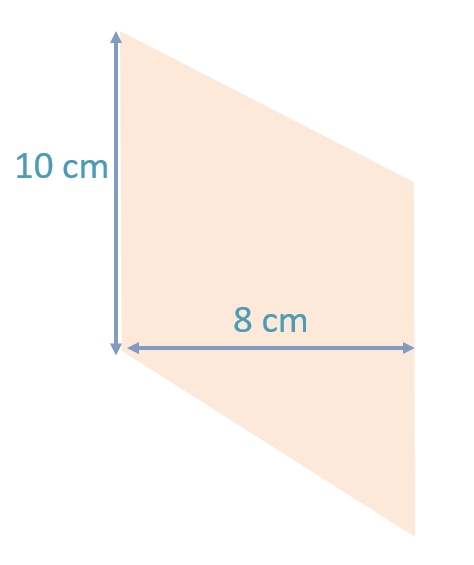The base is chosen as the known outer side length. The base in this example is 10 cm. We choose this even though it is not on the bottom of the parallelogram.

The height is then the length between this base side and the side opposite to the base. The height is 8 cm.

The area of a parallelogram is base x height.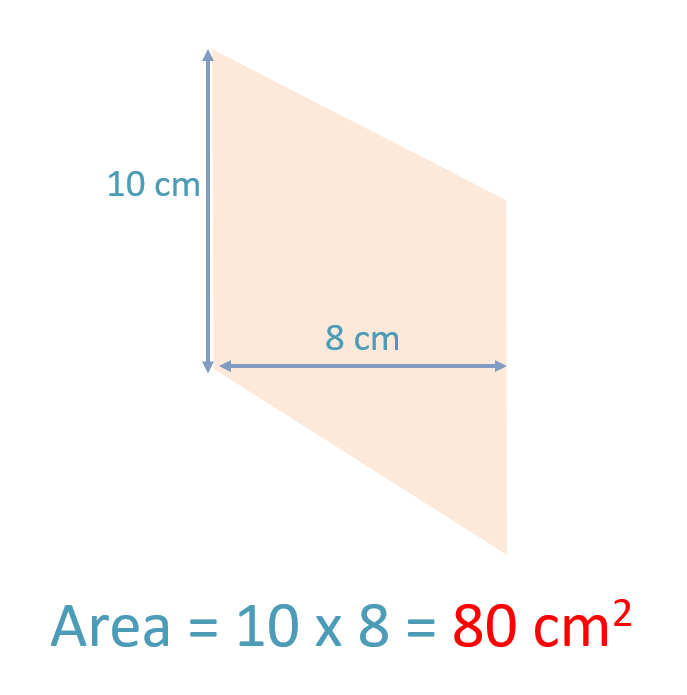The area is 10 cm x 8 cm = 80 cm2.

If a parallelogram is on its side, we can imagine it rotated around to see what its base would be.Now we can see the base and height more easily.

## What is the Formula for the Area of a Parallelogram?

The formula for the area of a parallelogram is A = b x h, where b is the base length and h is the perpendicular height. This formula can be written more simply as A = bh.

Here is an example of using the area of a parallelogram formula.

The base is 5 m and the height is 8 m.A = b x h.

b = 5 and h = 8, so A = 5 x 8 = 40.

Therefore the area of this parallelogram is 40 m2. Since we measured the sides in metres, the area is measured in metres squared.

Here is another example of using the formula to find the area of a parallelogram.

The base is 3 mm. The perpendicular height to this base is 7 mm. It is 7 mm from the base to the top of the parallelogram.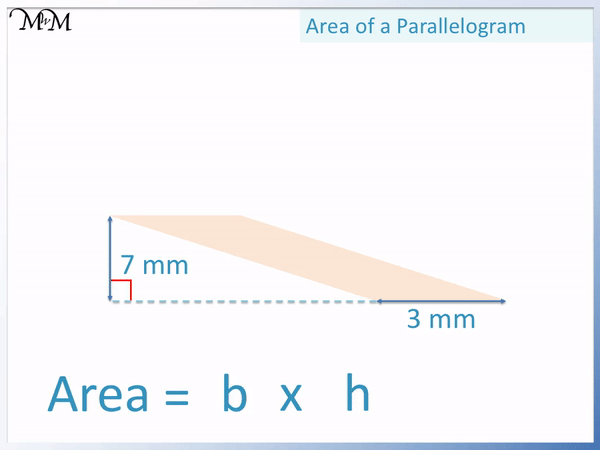A = bh. This means that we multiply b and h together.

A = 3 mm x 7 mm = 21 mm2.

This means that the area of this parallelogram is 21 mm2.

## Why is the Area of a Parallelogram Base Times Height?

The area of a parallelogram is base times height because it can be rearranged into a rectangle with the same area. A rectangle is a special type of parallelogram. The area of a rectangle is base times height and so, the area of a parallelogram is also base times height.

The area of a rectangle is found by multiplying the base by its height.

Each parallelogram can be rearranged to form a rectangle. We start by moving this marked triangle over to the right hand side.We now have a rectangle.The area of a rectangle is written as length x width or base x height.

Therefore the area of a parallelogram is also base x height.It is important to note that the base and height of a rectangle meet at right angles and so, the base and height of a parallelogram also need to meet at right angles in order to use the area of a parallelogram formula.

## A Rectangle a Type of Parallelogram

A rectangle is a type of parallelogram that has four right angles. A parallelogram is a shape with four sides and two pairs of parallel sides. A rectangle meets this definition and so, it is also a type of parallelogram.

A parallelogram is a type of quadrilateral, which means that it has four sides.

A parallelogram is called a parallelogram because it has two pairs of parallel sides.A rectangle is a special type of parallelogram because it has four sides and two pairs of parallel sides.Now try our lesson on Area of a Compound Shape where we learn find the area inside a shape made up of more than one rectangle.